Binary Numbers and the Working of Computers# Binary Numbers and the Working of Computers##### Udayan Shakya

|

9 min read

While possessing a vast knowledge of mathematics is not necessary to learn computer programming, it certainly helps to have a basic grasp of some of the foundational mathematics that makes computing possible. And what could be more fundamental to modern computing than binary mathematics?

The term 'binary' means something that has only two possible objects or states. In the binary number system, these two objects are the numbers 0 and 1. These two numbers can represent a variety of things.

For example, in computer logic, 0 represents "false" while 1 represents "true". Or they could be used to represent ordinary numbers as combinations of 1's and 0's. An example of this would be the representation of the numbers 0, 1, 2, 3, and 4 in three binary digits as 000, 001, 010, 011, and 100 respectively.

But what does this all mean at the fundamental level of computing? Why is the binary number system used as the foundation for all of our computing?

Perhaps it would be easier to understand all of this if we can understand the basic working of computers at the machine level.

### 0's and 1's: Interpreting the Workings of Computer Circuitry

computers operate on the electrical signals generated by these circuits. In order to design a computer that runs efficiently, we need a system that can interpret electrical signals in a simplified and effective manner.

A good way of doing this is to interpret electrical signals as binary values: 0 for a low voltage value and 1 for a high voltage value. An easier way of thinking about this is to imagine a light bulb. If the bulb is off, that state is interpreted as having the value 0. If it is on, it is interpreted as having a value of 1.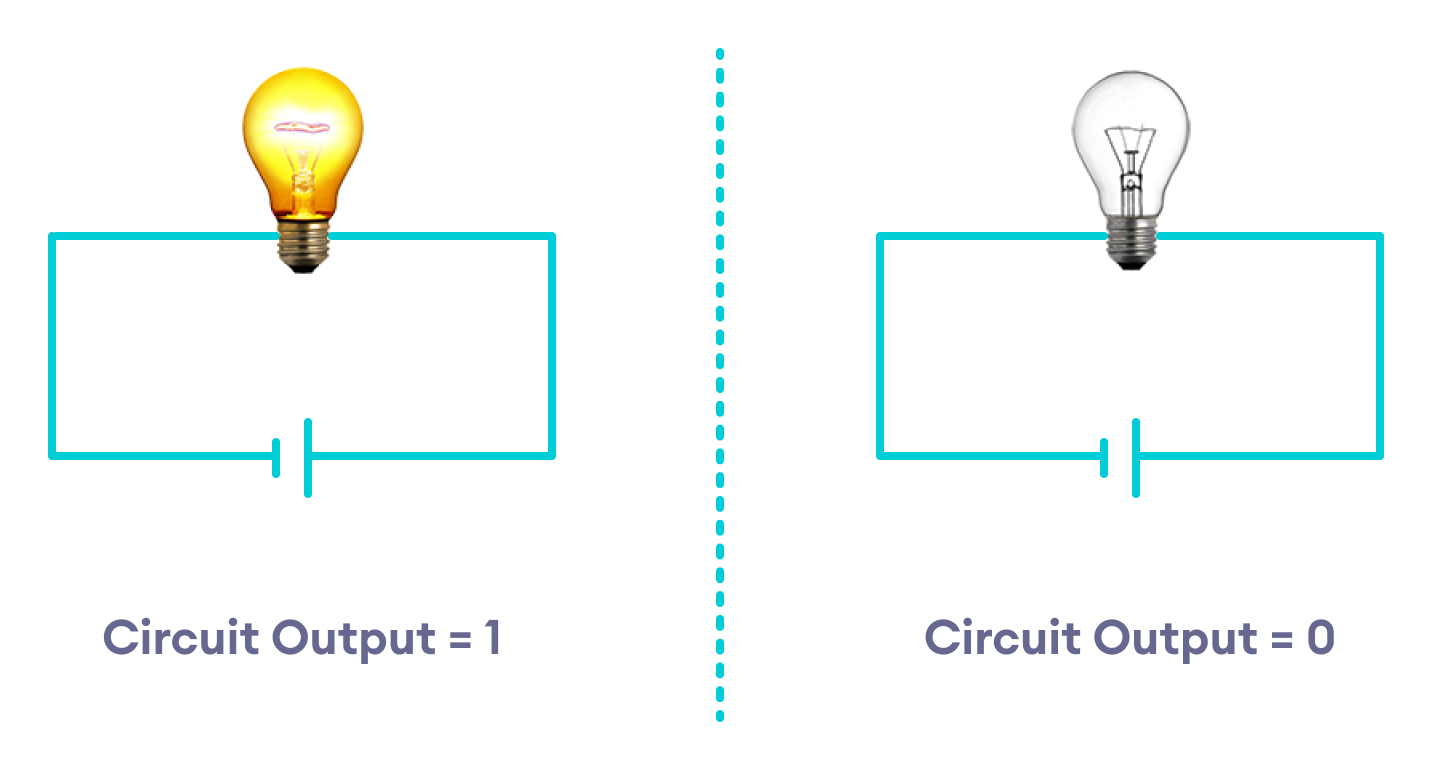Interpreting Bulb States in Binary

This broad generalization reduces the range of interpretation of each electric signal into two distinct values, instead of an infinite range of continuous voltage values.

With this method of operating and interpreting electronic circuits in place, we can proceed to design coded systems based on the binary bits to aid in our computational tasks. These systems could be binary logic (restricted only to true or false values), base-2 number representation of numerical values, or using other systems that rely on a series of binary numbers to represent text, pictures, or sounds.

Essentially, our computers use a series of high-voltage and low-voltage electrical signals (binary values) to represent everything from texts and numbers to images and sounds. There are special electronic circuits, such as flip-flops and other circuits, that can "store" or retain these specific patterns of electrical signals for extended use.

For instance, one flip-flop may have a number of inputs that currently has a high voltage output (which we interpret as 1). Suppose the next two flip-flops have low-voltage outputs 0. We could combine these three outputs to get a value of 100, which in binary is the same as the number 4.

Understanding binary numbers can thus help us understand some of the fundamentals of computer operations at a sufficiently abstract level, even though our feeble human intellect might never allow us to understand the full complexity of computer operations.

And that is just as well, for working with simplified and abstract conceptions of computer operations is more than adequate for us students of computer science. In the sections that follow, we will take a brief look at some of the different ways computers use binary symbols to perform some of its most fundamental operations.

### Boolean Logic: Using Binary Numbers to Understand Computer Logic

Computer programs use a very specific system of logic to carry out their instructions. This is known as Boolean logic, formulated by the English mathematician George Boole during the 19th Century.

Boole developed a system of arithmetical and logical operations that utilize the binary system of numbers. Boolean logic deals with only two possible values: true or false. True is represented by 1 and false is represented by 0. All logical operations result in only one of these two binary values.

Modern computers use this form of logic to make decisions all the time. These decisions result in our computers taking a particular course of action instead of another.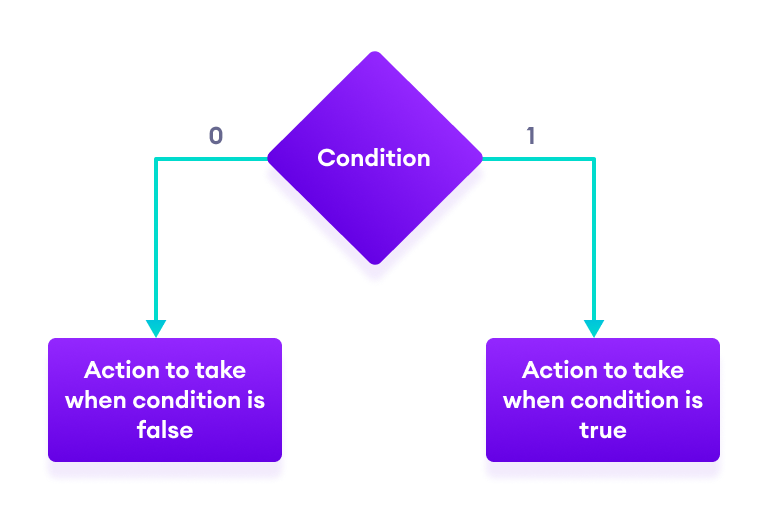Decision-making in Computers

To realize how crucial this system is for computers, one need not look any further than the existence of Logical Operators in most programming languages: the AND, OR, and NOT operators.

These operators are directly taken from the AND, OR, and NOT operations from Boolean logic. And anyone with a cursory knowledge of programming knows that these operations are central to programming.

But the influence of Boole's work doesn't end there. In fact, many programming languages have a data type named boolean, which can only store either "true" or "false" i.e. 1 or 0.

These boolean variables and logical operators are fundamental components used in implementing conditional statements and control statements in programming languages. As a result, their importance cannot be overstated, since this is Programming 101.

There are also many other, more creative, and more sophisticated ways we can use binary numbers in programming languages. However, this blog post serves as a mere overview of some of the things binary numbers can be used for.

As such, we won't be diving into any technical programming details. Perhaps I will explore more of those topics in later blog posts. For now, let us just explore the simpler topic of numerical representations in computers.

### Representing Numerical Values in Base-2

Numerical values are represented in our computer systems in some form of the base-2 number system. The normal numbers we use in everyday life is the base-10 number system. For instance, the number 135 gives us a value of one hundred and thirty-five.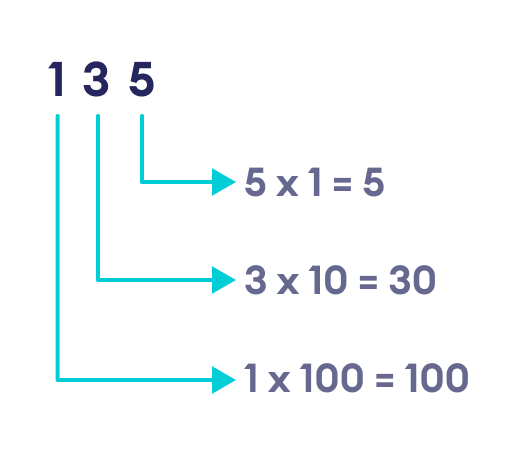Decimal Place Values

As we can see from the image above, each digit starting from the right side is multiplied by increasing powers of 10, starting with (10 ^ 0 = 1), then (10 ^ 1 = 10) and finally (10 ^ 2 = 100). The more the digits, the more the succession of powers of 10. This is the reason why this number system is called the base-10 number system.

The base-2 number system works in the same way, except that we multiply each bit (a binary "digit") with successive powers of 2. As an example, let us take the base-2 number 1011 and see what base-10 number it represents.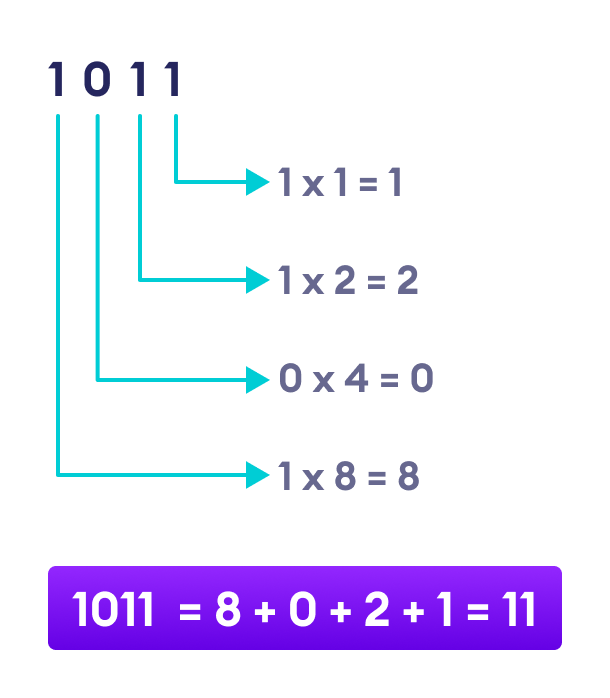Binary Place Value

As we can see, the binary number 1011 is equivalent to the number eleven (11) in base-10.

Of course, the way binary numbers are grouped also matters. We know that 1011 represents the number 11 in base-10. But what if we grouped the same series of bits as 10 11? Are they two different numbers 10 and 11? Or are they a single number 1011?

This shows us the importance of the way we group our numbers. And in computers, the numbers are grouped in a lot of different ways. For instance, the integer data type int in C++ stores a single number in a series of 32 binary numbers. So the base-10 number 0 is represented by a series of 32 zeroes, while the number 1 is represented by 1 preceded by 31 zeroes to its left.

### Textual and Character Representation

We have seen that the base-2 number system forms the basis of numerical representation in our electronic devices. And while this is true for systems of textual representation, their workings are quite different.

The most common systems for representing characters are ASCII (American Standard Code for Information Interchange) and Unicode (which is an extension of ASCII). These systems assign unique numeric values to characters and store them in binary format.

For instance, the ASCII system originally used 7 bits to represent a character. Currently, that has been extended to 8 bits. As an example, the character A is represented by a numeric value of 65 in ASCII. The binary for 65 is 1000001. Notice that the binary representation consists of 7 bits. In extended ASCII, this will be stored as 01000001 so that the total number of bits is 8.

Similarly, the ASCII code for the character a is 97, and it is represented by 01100001 in extended ASCII. There are also very special characters that are represented by 0, 1, 2 and so on. And they are represented as 00000000, 00000001, 00000010 and so on.

This system serves well for representing English and certain European characters and symbols, but it is woefully inadequate for representing symbols from languages across the world. In order to accommodate further symbols, Unicode was developed.

Unicode originally used 21 bits per symbol as opposed to the 7 bits originally used by ASCII. This greatly expands the range of values that can be used to represent characters. The original ASCII codes are accommodated within the Unicode system.

Currently, an encoding system based on Unicode, called UTF-8, is the most commonly used encoding system in web applications. The UTF-8 can use up to 32 bits per symbol, which means it can represent an even larger variety of characters.

### Pixels and Images

Unsurprisingly, images are also frequently represented by numbers. In computers, images are most commonly made with the help of tiny colored squares called pixels. Think of a mosaic in real life: an image or pattern is made by combining together numerous small, colored pieces. Or a jigsaw puzzle, where we combine smaller pieces to create a bigger, complete image.

Pixels work in a similar way. Thousands of tiny colored squares make up the images that are displayed on our screens. There are many ways the colors in the pixels are coded, but the most commonly used code is the RGB code (Red, Green, Blue).

RGB codes work by combining red, green, and blue colors to produce all the shades of colors we see in our modern devices. Each of the three color components is codified by a number, whose values range from 0 to 255. Thus, there are three sets of numbers that describe a pixel.

As an example, let us consider the color represented by the RGB code (142, 150, 123). This color code has 3 components: Red = 142, Green = 150, and Blue = 123.

Inside our computers, each of these color components are represented by their binary equivalents using 8 bits, and then combined together. For example, the binary for 142 (Red component) is 10001110, the binary for 150 is 10010110, and the binary for 123 is 1111011.

Red = 142 = 10001110

Green = 150 = 10010110

Blue = 123 = 01111011

The computer combines these numbers from left to right to store the RGB code in its memory.

Complete RGB code = 100011101001011001111011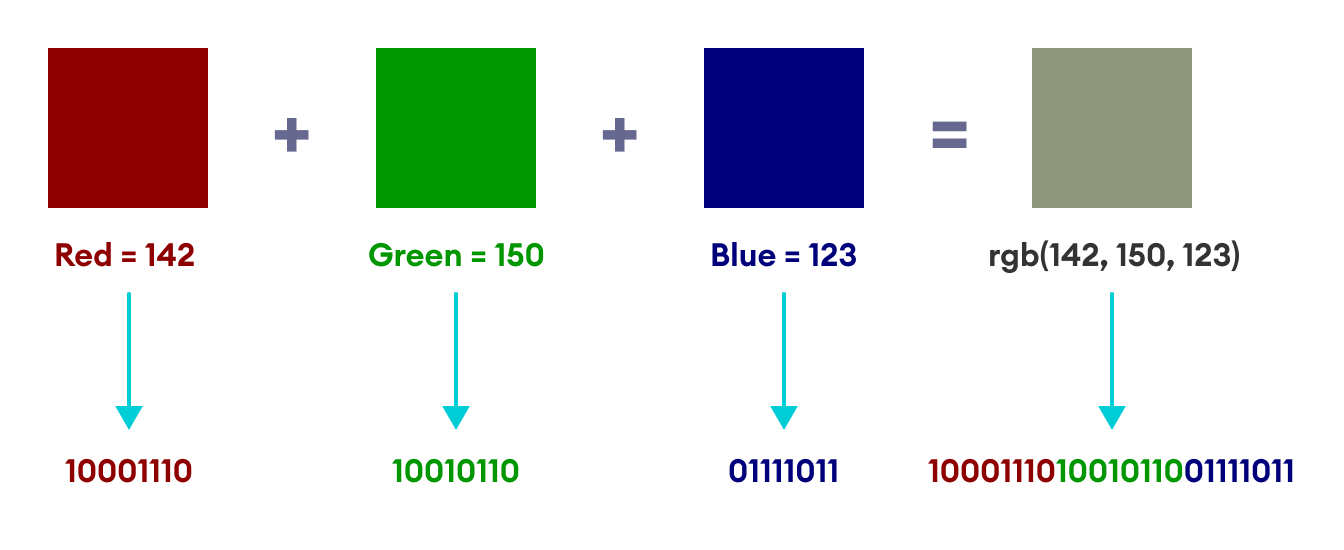Working of RGB Code

Thus, we can see that even images are represented in binary. In fact, the knowledge of the binary codes of image pixels opens the doorway for fun little applications of image manipulation, such as hiding one image inside another. I have already written about a simple method of image hiding in my previous blog Steganography: Hiding Information Inside Pictures. You can check it out for an actual practical application of binary numbers in computer science.

### Final Thoughts and Takeaways

Binary numbers form one of the core foundations of modern computing. And while we have briefly glanced over some of the ways our computers use binary numbers, we have left out a lot as well, since an exhaustive list of applications and explanations is beyond the scope of this blog.

That being said, we have covered quite a few interesting topics here, basic though they may be. We have seen that

• physical activities of the computer circuitry are interpreted using binary notation i.e. in a single circuit, low voltage values are interpreted as 0 and high voltage values are interpreted as 1.
• computers use Boolean Logic by interpreting 1 as true and 0 as false while performing logical operations.
• numbers are represented in base-2 format in varying groups of bits according to the application (some numbers are represented by 16 bits, others by 32 bits, and some others by 64 bits).
• characters and text are assigned unique numerical values, which are then converted to base-2 format in groups of either 7 or 8 bits (ASCII) or 32 bits (UTF-8).
• images are represented by groups of tiny colored squares called pixels, each of which is coded in numbers that are eventually converted to binary

And that wraps up this blog, and I hope I have shed some light on why binary numbers are so important to computing.##### Udayan Shakya

Udayan is a physics graduate and works as a content writer at Programiz. When he's not busy with programming, he passes his time consuming the books he possesses in his small library.